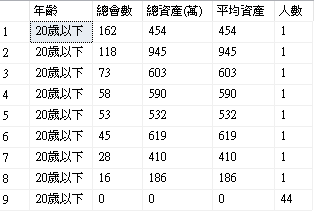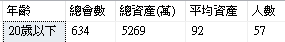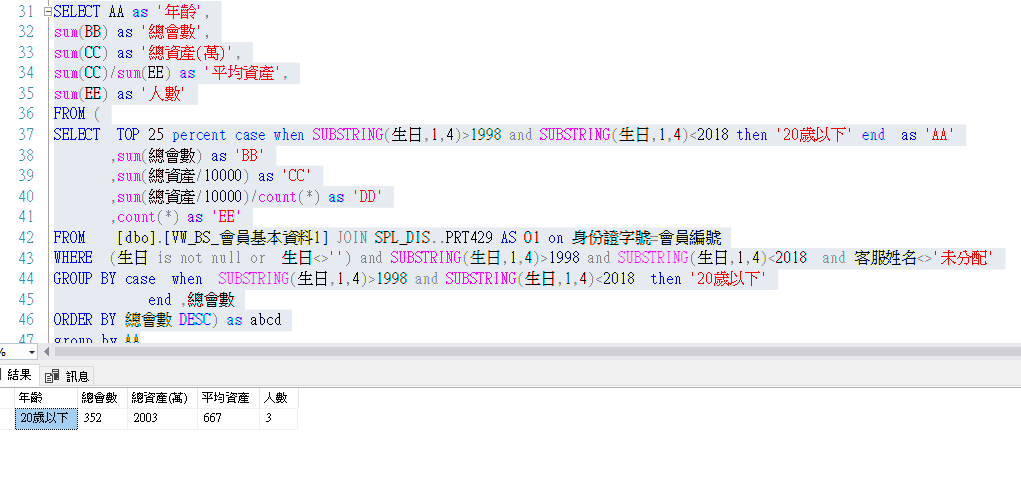#0

## 請問如何抓出 '20歲以下' 佔總會數前25% 的所有總會數跟總資產和平均資產和人數?

SELECT TOP 25 percent case when (SUBSTRING(生日,1,4)) BETWEEN 1998 AND 2018 then '20歲以下' end as '年齡'
,sum(總會數) as '總會數'
,sum(總資產/10000) as '總資產(萬)'
,sum(總資產/10000)/count() as '平均資產'
,count(
) as '人數'
FROM [dbo].[VW_BS_會員基本資料1] JOIN SPL_DIS..PRT429 AS O1 on 身份證字號=會員編號
WHERE '年齡' IN ( SELECT '年齡'
FROM [dbo].[VW_BS_會員基本資料1] JOIN SPL_DIS..PRT429 AS O2 on 身份證字號=會員編號
WHERE o2.總資產 = o1.總資產
ORDER BY [總資產] DESC)
AND (生日 <> null or 生日<>'') and (SUBSTRING(生日,1,4))>1998 and (SUBSTRING(生日,1,4))<2018 and 客服姓名<>'未分配'
GROUP BY case when (SUBSTRING(生日,1,4)) BETWEEN 1998 AND 2018 then '20歲以下' end ,總資產
ORDER BY 總會數 DESCdebbie5 iT邦新手 5 級 ‧ 2018-11-20 08:50:49 檢舉

### 1 個回答

1

1.假設原 SQL 是對的
2.其中"平均資產"改用 sum(CC)/sum(EE),而未使用 sum(DD) <= 可能不合理

SELECT AA as '年齡',
sum(BB) as '總會數',
sum(CC) as '總資產(萬)',
round(sum(CC)/sum(EE),0) as '平均資產',
sum(EE) as '人數'
FROM (
SELECT TOP 25 percent case when (SUBSTRING(生日,1,4)) BETWEEN 1998 AND 2018 then '20歲以下' end as 'AA'
,sum(總會數) as 'BB'
,sum(總資產/10000) as 'CC'
,count() as 'EE'
FROM [dbo].[VW_BS_會員基本資料1]
...略
ORDER BY 總會數 DESC
) AS TempTable
where 1=1
group by AA
having sum(EE) >0

rogeryao iT邦高手 1 級 ‧ 2018-11-20 09:15:25 檢舉

1.以下應該可去除
'年齡' IN ( SELECT '年齡'
FROM [dbo].[VW_BS_會員基本資料1] JOIN SPL_DIS..PRT429 AS O2 on 身份證字號=會員編號
WHERE o2.總資產 = o1.總資產
ORDER BY [總資產] DESC)
2.

3.
case when (SUBSTRING(生日,1,4)) BETWEEN 1998 AND 2018 then '20歲以下' end

case when SUBSTRING(生日,1,4)>1998 and SUBSTRING(生日,1,4)<2018 then '20歲以下' end

debbie5 iT邦新手 5 級 ‧ 2018-11-20 09:49:24 檢舉

rogeryao

rogeryao iT邦高手 1 級 ‧ 2018-11-20 10:41:03 檢舉

rogeryao iT邦高手 1 級 ‧ 2018-11-20 20:29:42 檢舉

1.因為你已經下好 SQL (類似明細表)才要做 sum ,大概就是這樣解.
2.可能還有一種解法更好,就是把你原本的 group 跟 sum 去除 , 只在最外層做一次 group 跟 sum ;這樣就不用做兩次 group 跟 sum

debbie5 iT邦新手 5 級 ‧ 2018-11-21 11:54:36 檢舉

## rogeryao 非常的感謝你哦~ 我昨天有做出來了